# 月之暗面

The DARKSIDE of the Moon

0%

# 回顾热噪声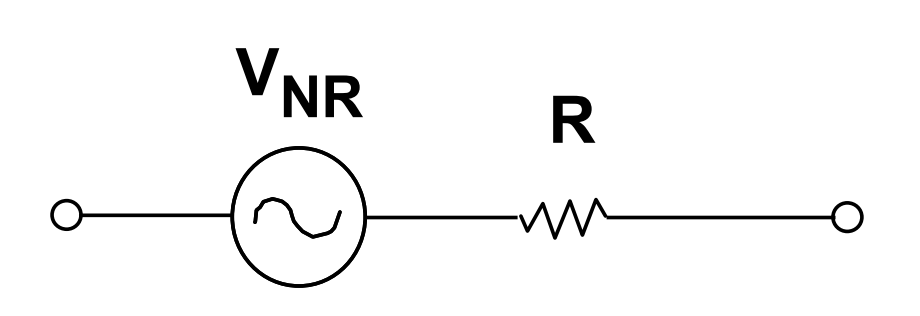$V_{Noise} = \sqrt{4kTR} \ \ (V/\sqrt{Hz})$

$V_{Noise} = \sqrt{4kTBR} \ \ (V)$

# 超越噪声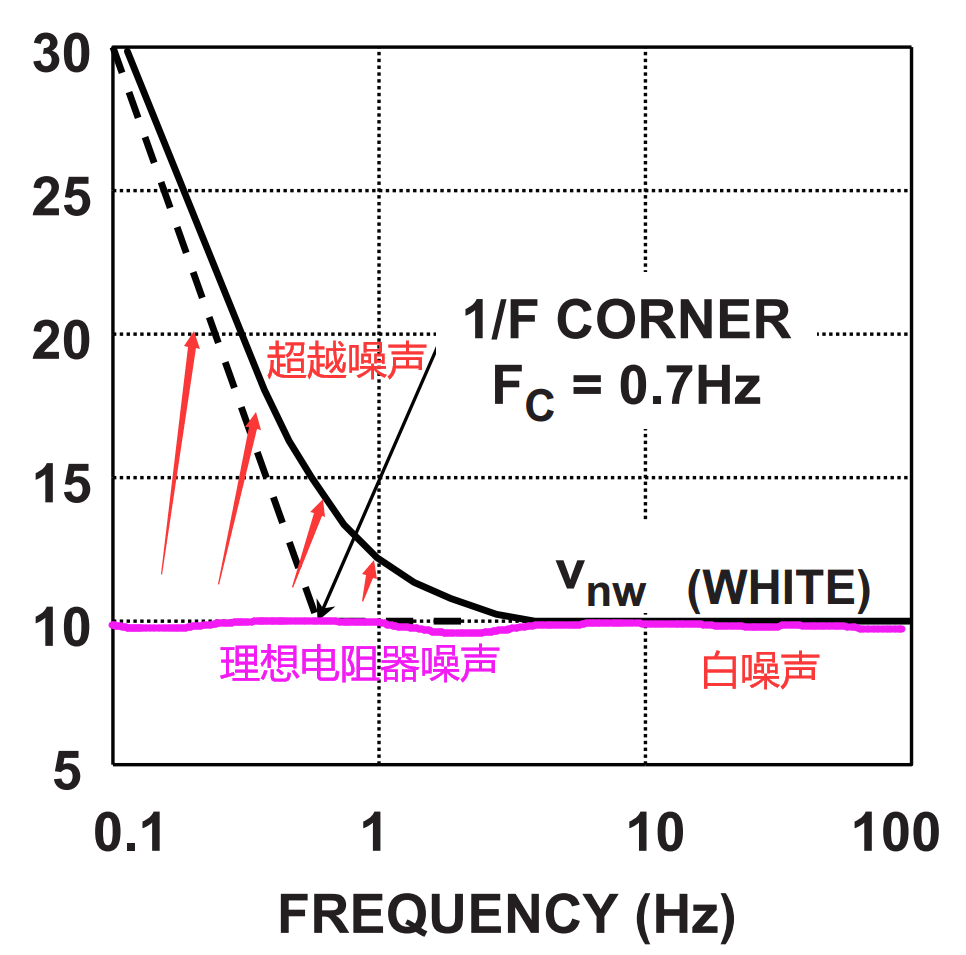# 运算放大器噪声源

• 以差分形式出现在输入端的电压噪声源
• 输入端的电流噪声源
• OpAmp外围电阻的热噪声

## 电压噪声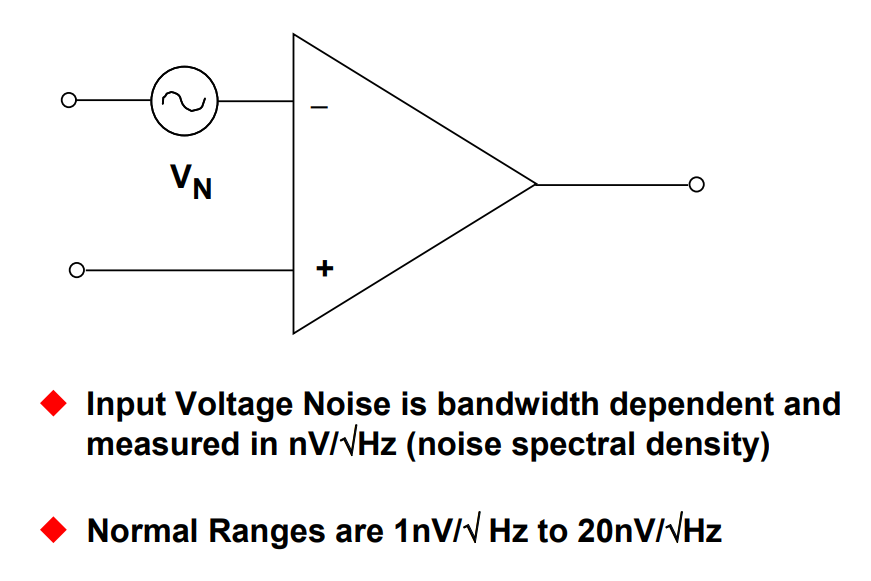OpAmp的输入电压噪声以噪声谱密度表示,单位通常是 $$nv/\sqrt{Hz}$$。这个参数通常会标注在器件的数据手册中，其大小因器件而异。通常所说的低噪声运算放大器的输入电压噪声密度在 $$10nV/\sqrt{Hz}$$ 以下，最低的能做到 $$0.9nV/\sqrt{Hz}$$。而通用运放的噪声可能要大得多，10几甚至几十都是有可能的。但总的来说，现代OpAmp的电压噪声水平基本在这个数量级上，不会有很大的跨度。

## 电流噪声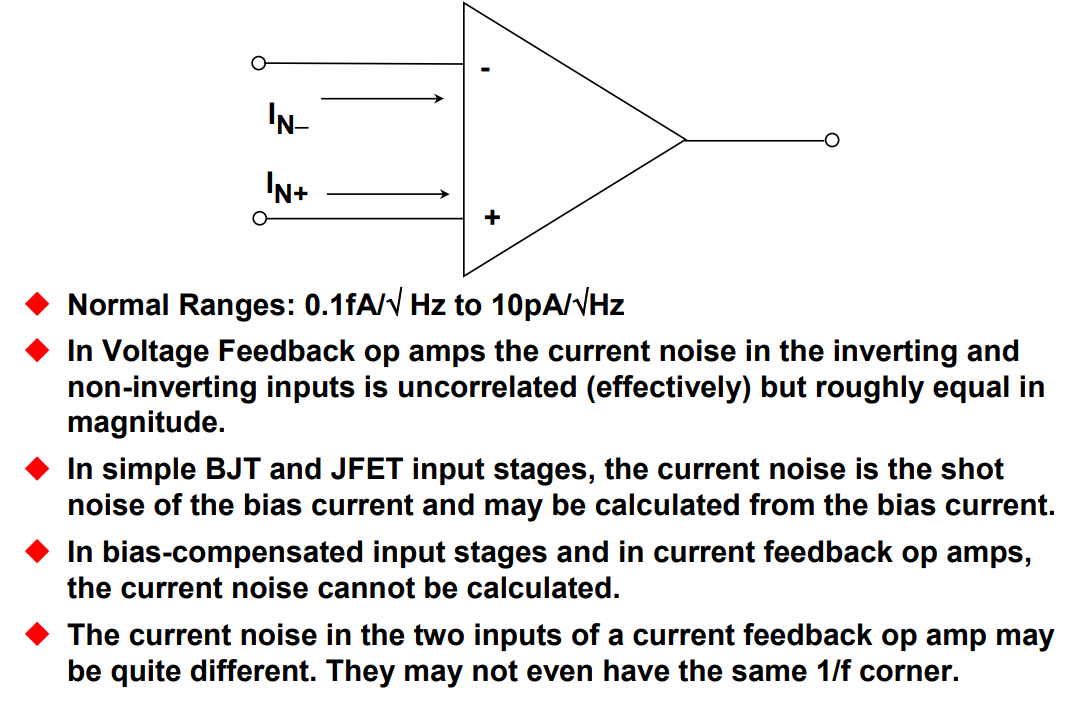## 合并上述噪声源

$V_{total} = \sqrt{V_1^2 + V_2^2 + V_3^2}$

## 单极点运算放大器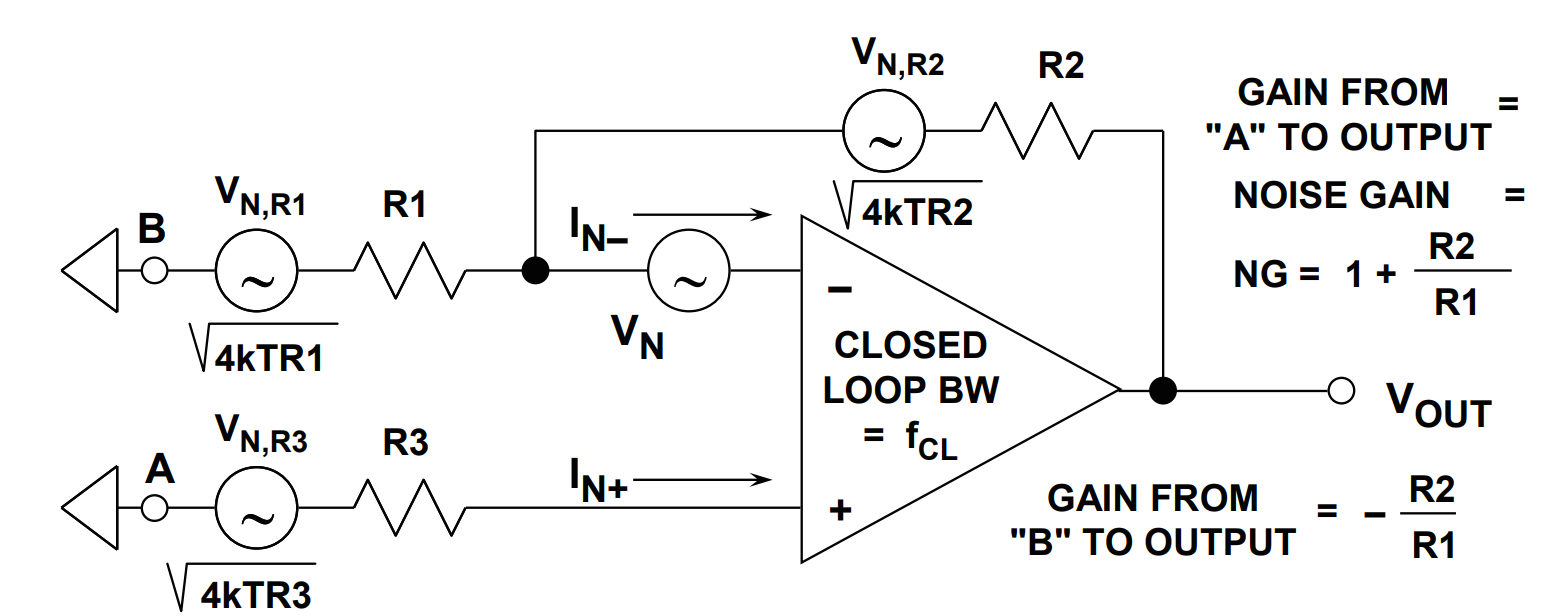• 3个电阻的约翰逊-奈奎斯特噪声(热噪声)
• OpAmp输入电压噪声
• OpAmp两端输入电流噪声

$G = -{R_2 \over R_1} \cdots 从A至输出\\ NG = 1 + {R_2 \over R_1} \cdots 从B至输出$

R3的热噪声:$$\sqrt{4kTR_3}$$ $$NG = 1+{R_2 \over R_1}$$

R1的热噪声:$$\sqrt{4kTR_1}$$ $$G = -{R_2 \over R_1}$$
R2的热噪声:$$\sqrt{4kTR_2}$$ 1

$V_{Noise} = BW \cdot \sqrt{4kTR_3\cdot (1+{R_2\over R_1})^2 + (I_{N_+}R_3)^2\cdot (1+{R_2\over R_1})^2 + \\ V_N^2\cdot (1+{R_2\over R_1})^2 + 4kTR_1 \cdot {R_2^2 \over R_1^2} + 4kTR_2 + (I_{N_-}R_2)^2} \\ BW = 1.57\cdot f_{CL}$

## 等效噪声带宽(ENB)

$ENB = \Delta f = {1\over 2\pi} \int_0^\infty |{H(\jmath\omega) \over H(max)}|^2 d\omega$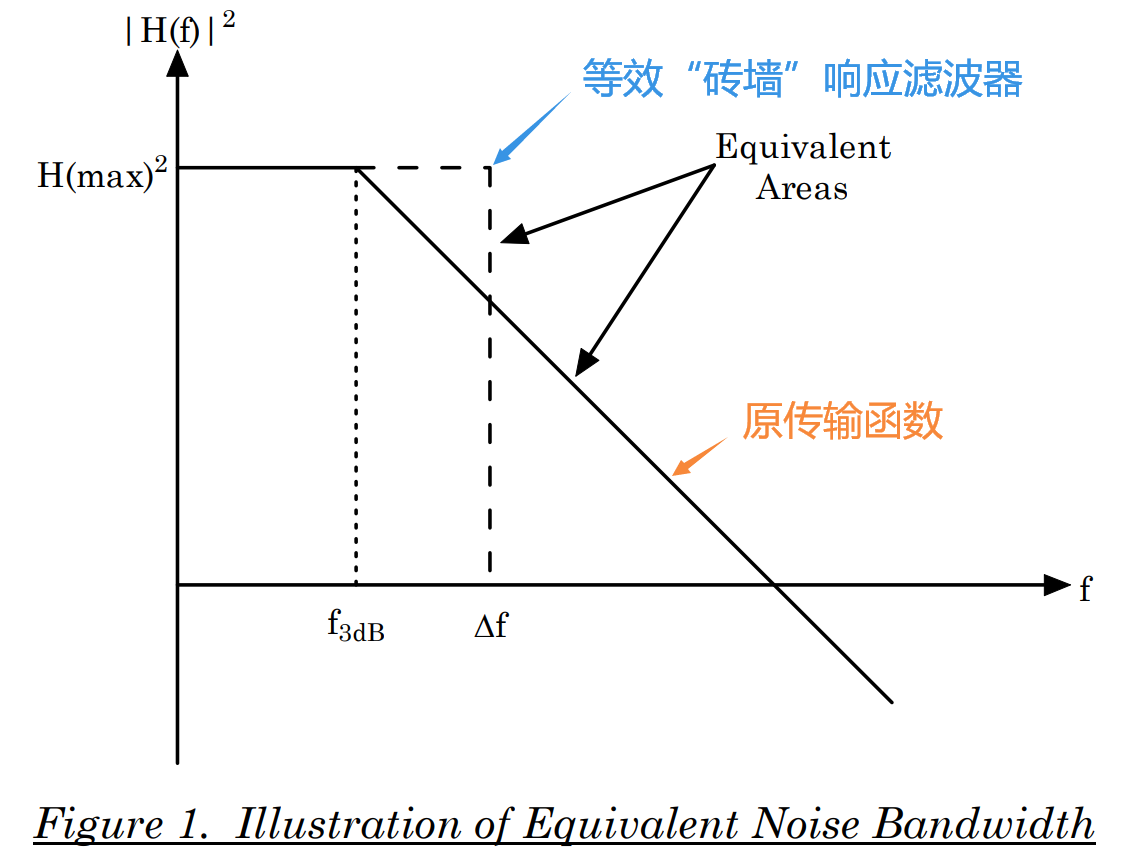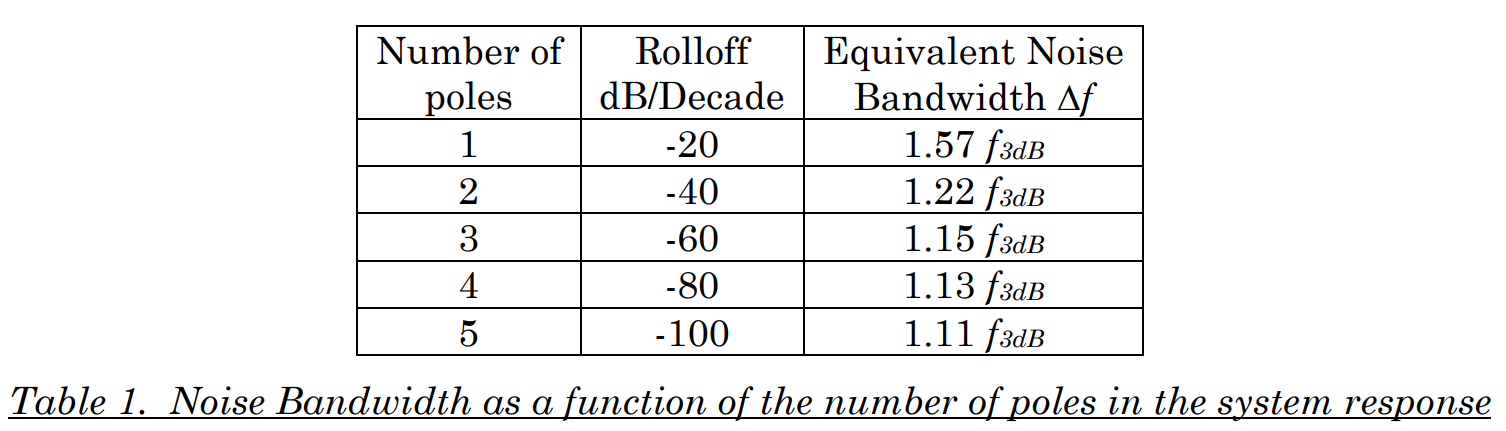• 如果低通滤波器是砖墙式响应,那么截止频率以上所有频率处的能量都为0;
• 如果低通滤波器不理想，即使是截止频率以上的频率分量仍存有残留能量;

# 利用仿真工具对噪声进行仿真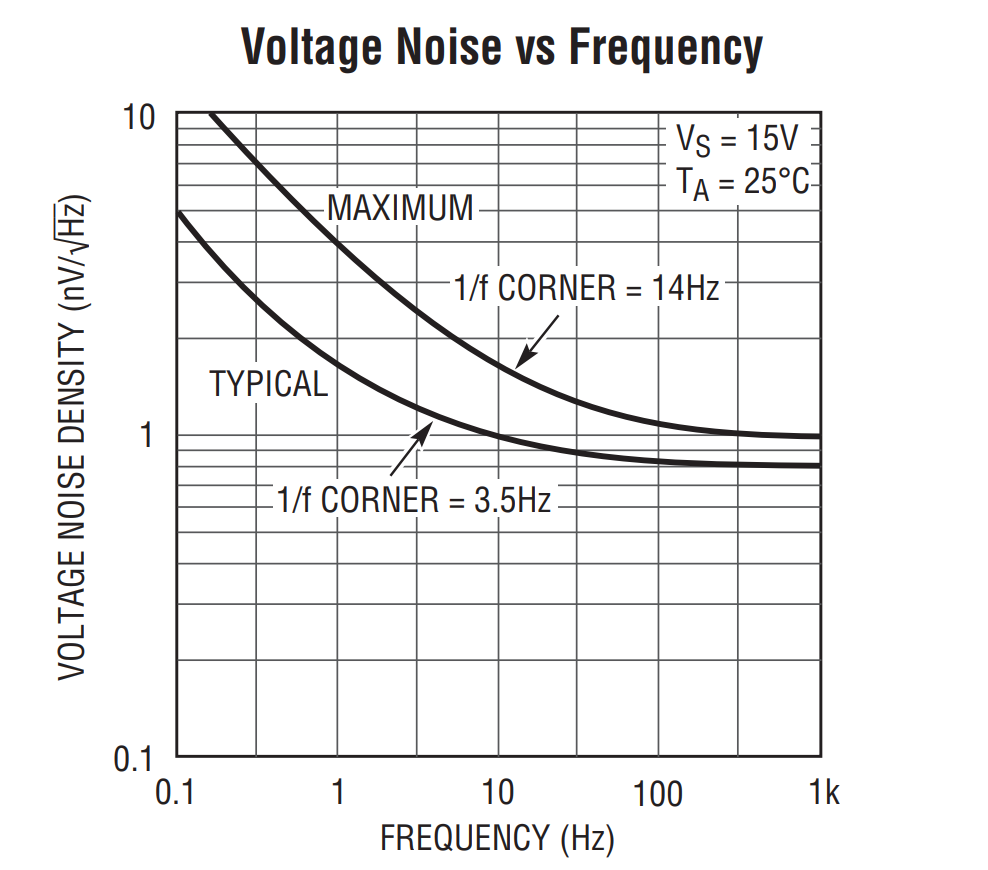.noise V(<out>[,<ref>]) <src> <oct,dec,lin> <Npoints> <StartFreq> <EndFreq>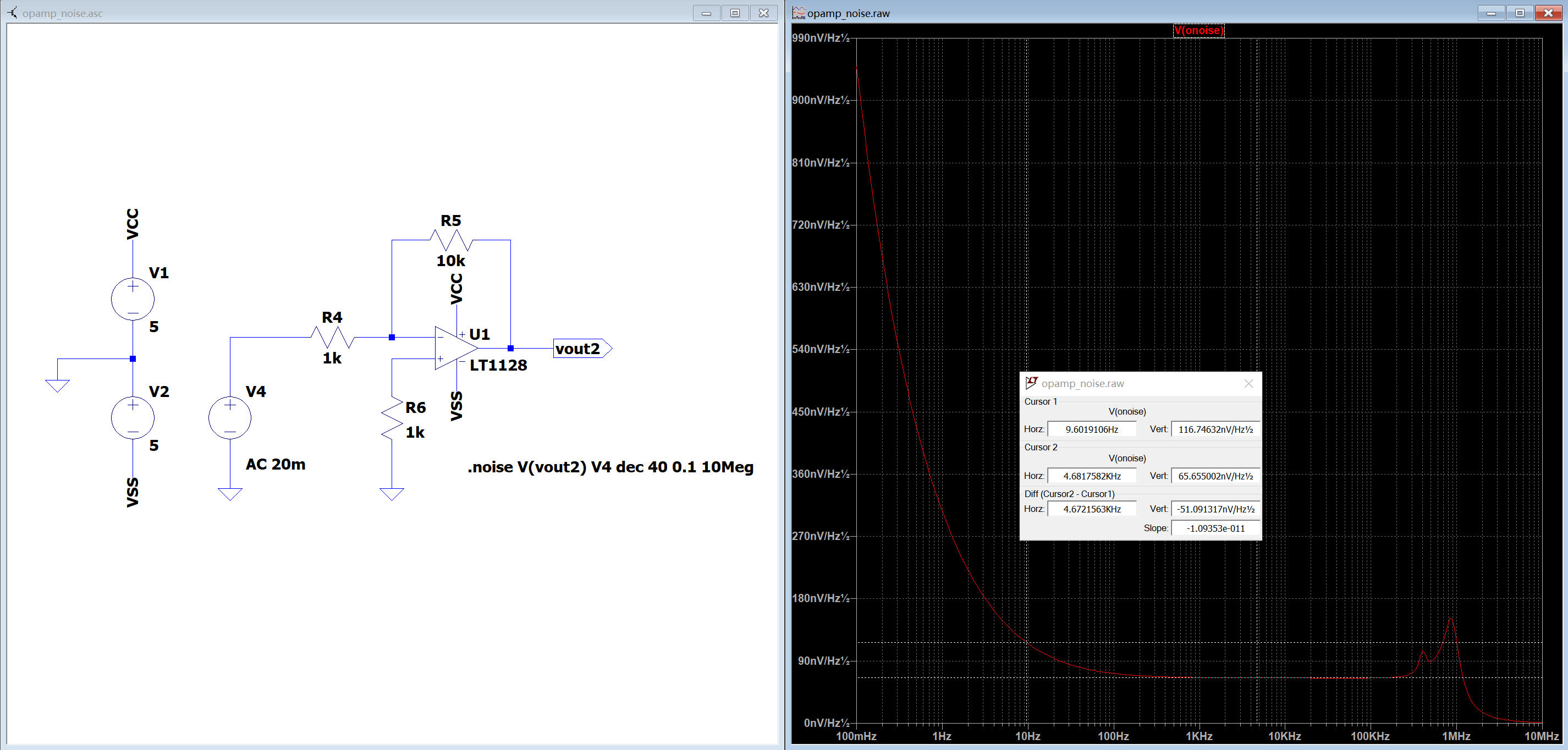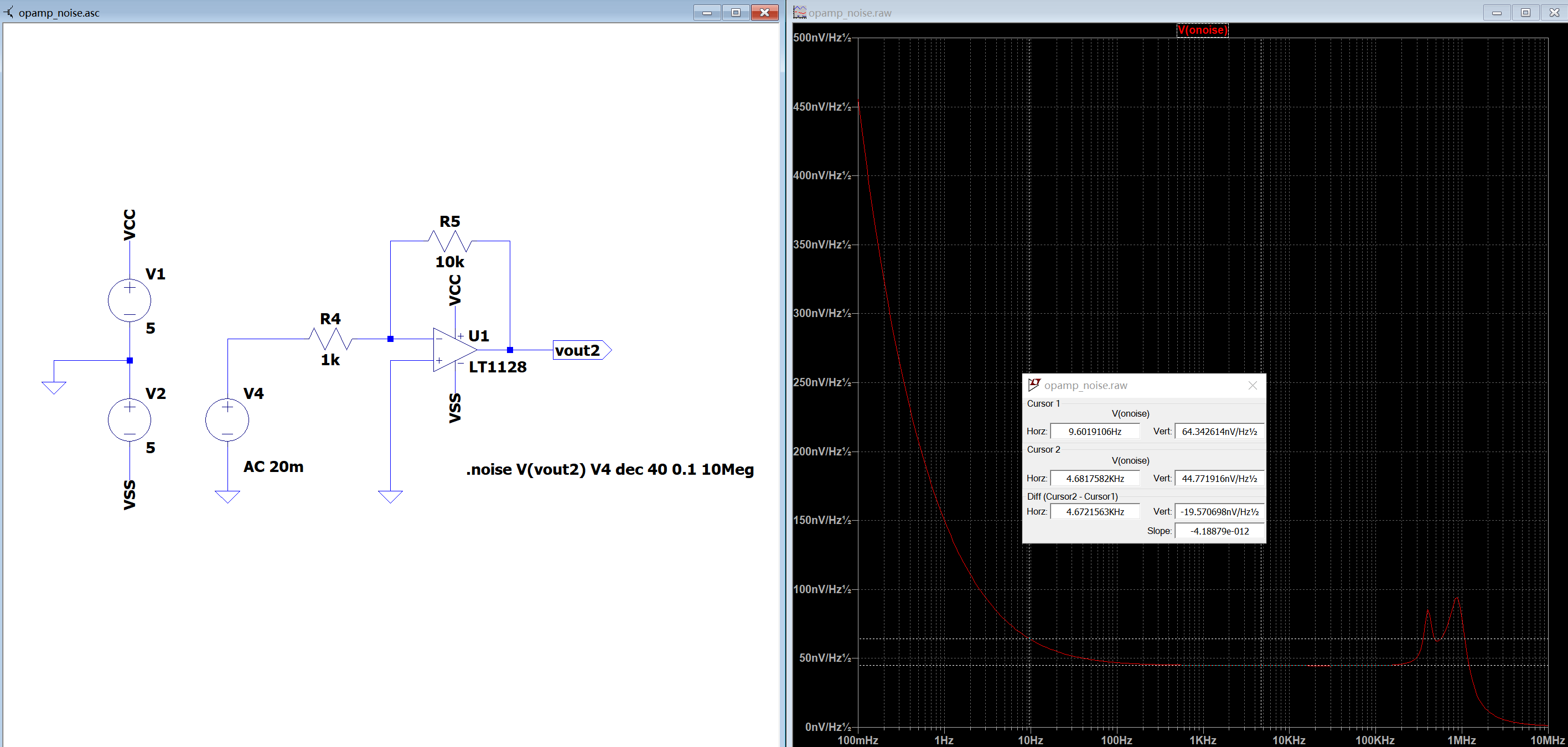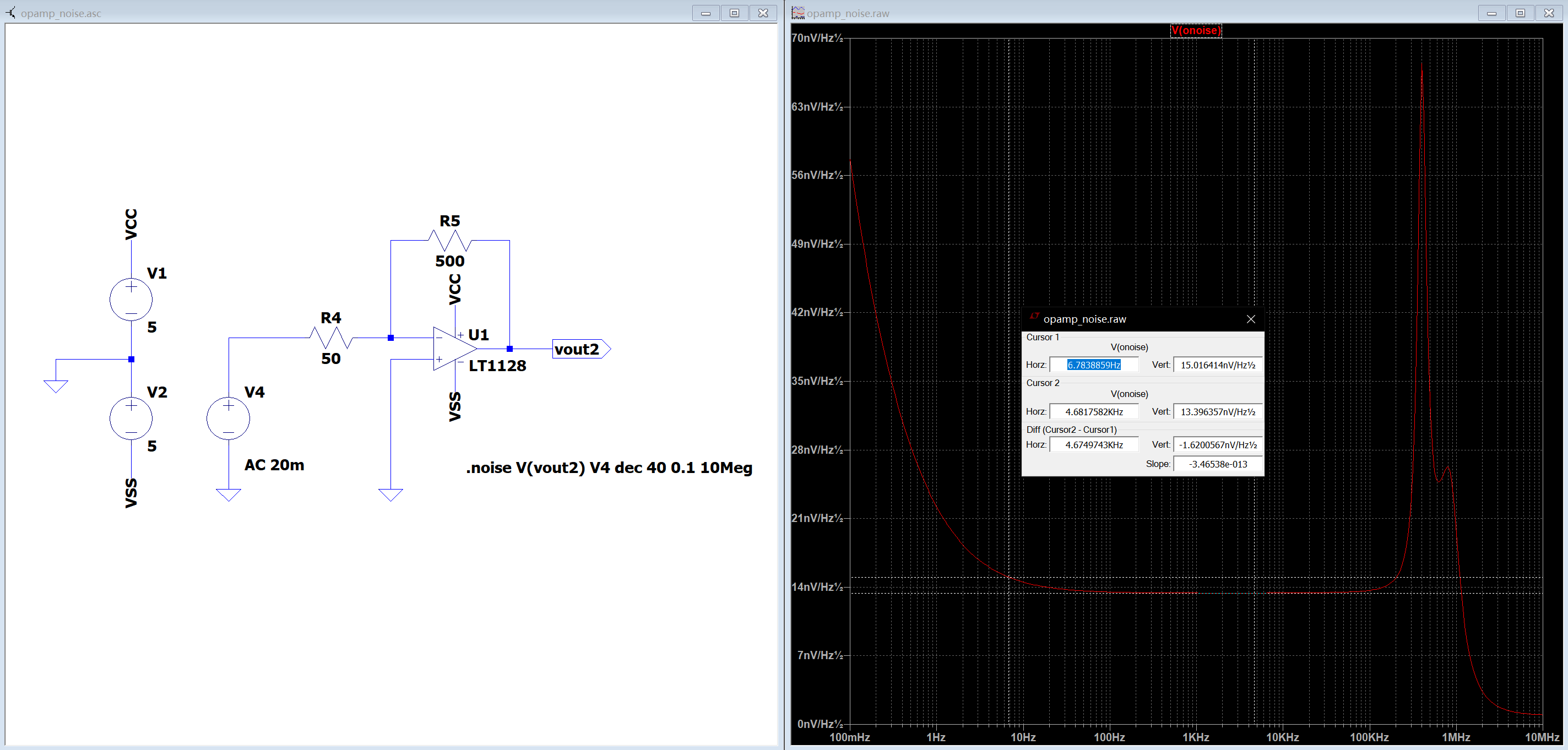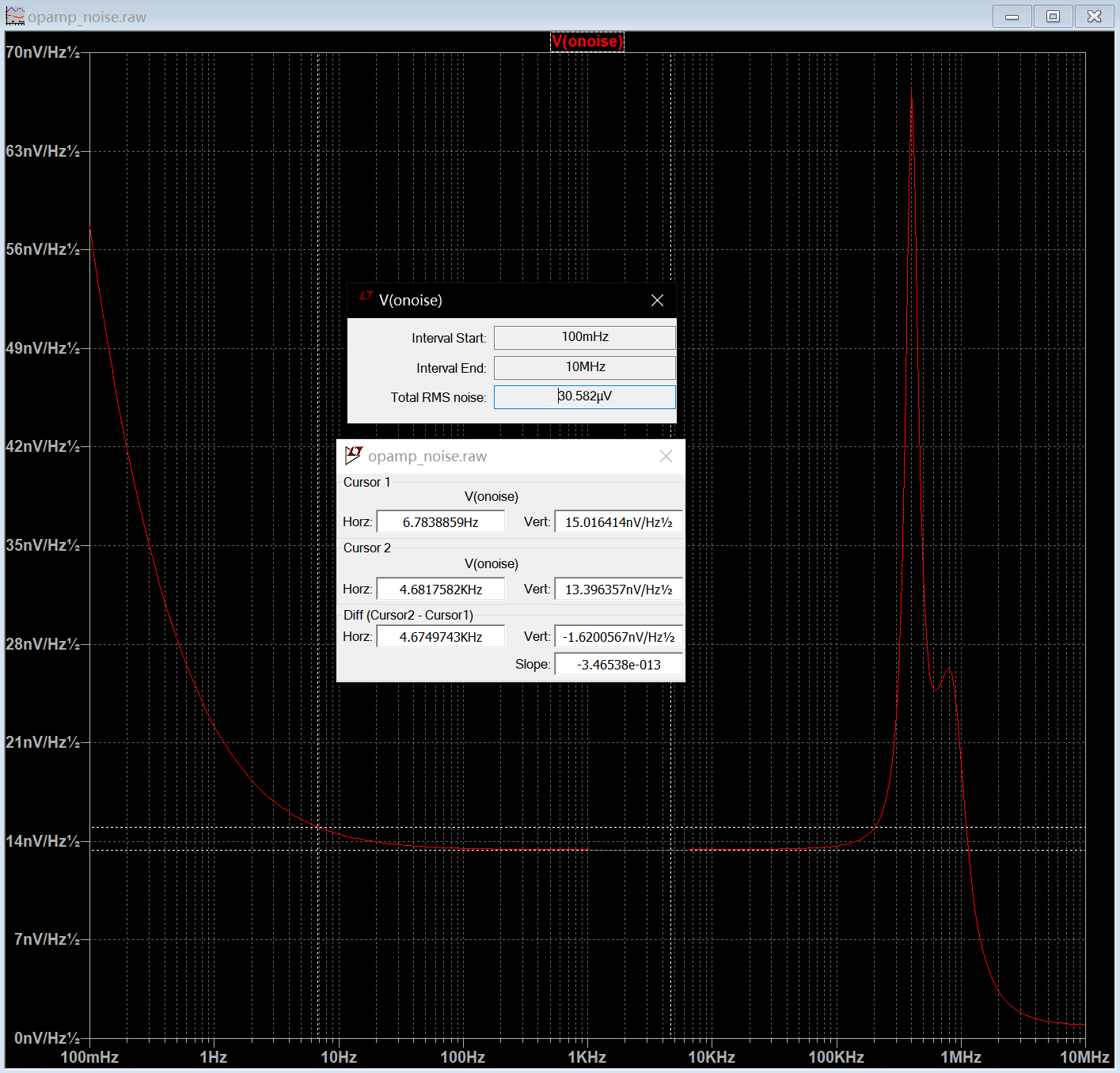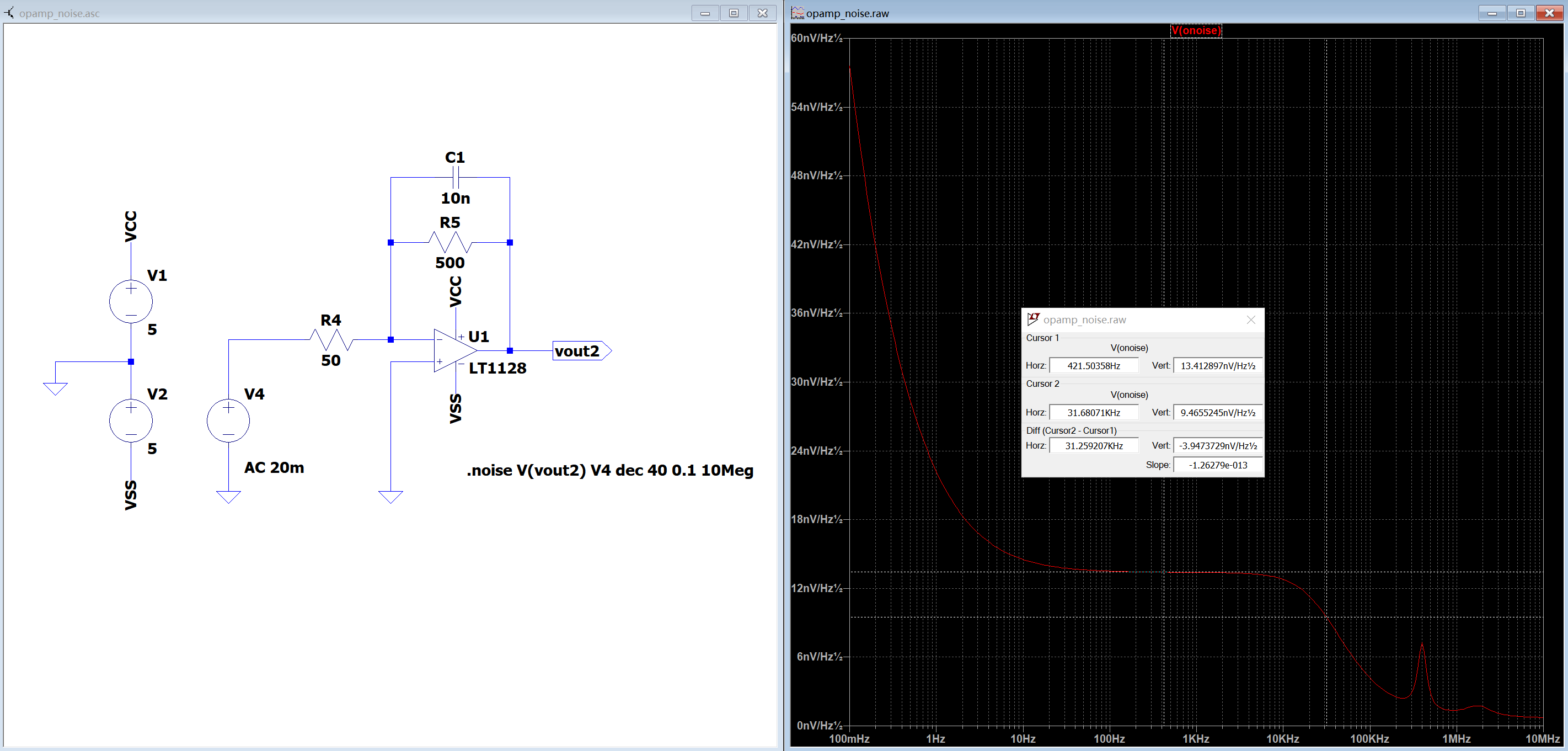# 噪声的统计性质